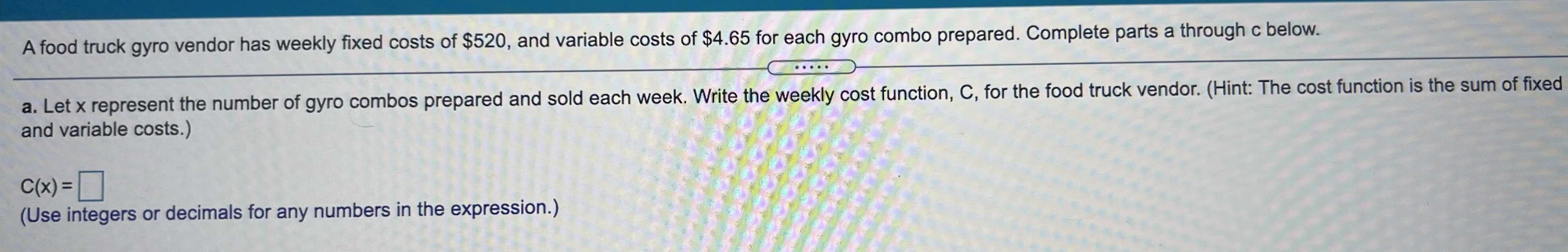### ¿Todavía tienes preguntas de matemáticas?

Pregunte a nuestros tutores expertos
Algebra
PreguntaA food truck gyro vendor has weekly fixed costs of $$\ 520$$ , and variable costs of $$\ 4.65$$ for each gyro combo prepared. Complete parts a through c below. a. Let $$x$$ represent the number of gyro combos prepared and sold each week. Write the weekly cost function, C, for the food truck vendor. (Hint: The cost function is the sum of fixed and variable costs.)

C(x)=______?